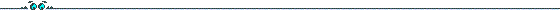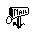## Hyperelliptic Curves Allowing Fast Arithmetic### Introduction

Besides RSA most public key cryptosystems are based on the discrete logarithm problem or related problems as underlying one-way function. To set up such a system one needs a cyclic group and a generator. Common choices are a subgroup of the multiplicative group of a finite field or the group of points on an elliptic curve. In 1989 Koblitz proposed the use of the Picard group of hyperelliptic curves over a finite field as a further group for cryptographic use. All the cryptosystems generalize obviously to this group.

We consider hyperelliptic curves over a field Fqn defined over the small field Fq. These subfield curves were first proposed by Koblitz for the case of elliptic curves. They offer advantages in the implementation of the cryptosystems since they allow faster computation of m-folds.
In the cases most suitable for applications q is fairly small, hence q=2,...,5 to obtain a large speed-up without storing too many elements. The genus should not be larger than 4 to avoid the index calculus attacks (see Pierrick Gaudry). To learn more about Koblitz curves consider the technical report by Christian Günther, Andreas Stein and myself. A shortened version of this appeared in: Proceedings of the Seventh Annual Workshop on Selected Areas in Cryptography, SAC 2000.

### Preprints and Thesis

have moved to a page of their own. The page contains several preprints on efficient and explicit arithmetic on hyperelliptic cuves especially of genus 2.

### Mathematical Background

In the following files you find complete lists of all classes of non-isogenous hyperelliptic curves of the indicated genera with the property that the characteristic polynomial of the Frobenius endomorphism is irreducible over the integers. Furthermore we only consider hyperelliptic curves with at least one Fq-rational Weierstrass point.

For odd characteristic a hyperelliptic curve of genus g is defined by an equation

v2=f(u),
where f(u) is a squarefree polynomial of degree 2g+1.
In characteristic 2 the curve is defined by
v2+g(u)v=f(u),
where deg f(u)=2g+1, deg h(u) <= g and the partial derivatives do not vanish simultaneously at any point of the curve over the algebraic closure.
The files are organized as follows: The first part of the files lists the (resp. both) defining polynomial(s) and the corresponding characteristic polynomial of the Frobenius endomorphism. In the second part the cardinality of the class group of suitable extensions is computed. The extensions are chosen in such a way that the class number is about 2160. Some of the files also contain the factorization of the class numbers.

To avoid the attack of Frey and Rück one has to avoid curves for which the cardinality of the field qn has a small order modulo the large prime l dividing the class number. Supersingular curves have the property that this order is always comparably small thus we did only compute the class numbers for non-supersingular curves. Notice that also for the cases listed in the files one needs to check that the group order is large, say larger than 2000/log2qn.

We did not consider the genus 4 case for q=4,5 since then the amount of precomputations is probably too large for small devices, the degree of extension gets small such that one needs to take care of Weil descent attacks and furthermore the inevitable factors in the class number have grown such that we usually lose too much when computing in these too large fields.
Be cautious when choosing curves defined over F4, the Weil descent attack applies also to hyperelliptic curves, (see Galbraith, Weil descent of Jacobians).

For two of the 6 classes of non-supersingular binary curves of genus two the class numbers have been published in the preprint of Günther/Lange/Stein.

Herewith these files are made public. They are freely available for research and educational purposes. I don't want to attach any legalistic licensing restrictions on the use of these curves.
However, I would appreciate to be informed of the usage of these curves in implementations.

### Programs

I wrote two programs to play around with these curves. They are written in Magma. If you find any bugs, please inform me!

• FrobSelf: Allows one to define a hyperelliptic curve and computes the characteristic polynomial of the Frobenius endomorphism. You can compute m-folds using the tau-adic method described above. To find curves with good properties, please consider the files below.
• FrobExample: Here we use the curve
C:v^2+(u^2+u+1)v=u^5+u^4+1

The class number over F289 is 2*191561942608242456073498418252108663615312031512914969, hence it is almost prime. You can compute m-folds using the tau-adic method. Furthermore we provide some routines to get timings.
FrobSelf:

for Magma 2.7
for Magma 2.8
for Magma 2.10

FrobExample:

for Magma 2.7
for Magma 2.8
for Magma 2.10

For reference implementation of the explicit formulae ask tanja@hyperelliptic.org.

### Tables with Examples

#### Files for curves over F2 (including factorization)

Note: The files presented here are simply text files. If you have any problems to obtain them do the following: move the mouse on the link, press the right button and chose "Save Link As" to download the file.

#### Files for curves over F5e-mail: tanja@hyperelliptic.org

back to Tanja Lange's Homepage